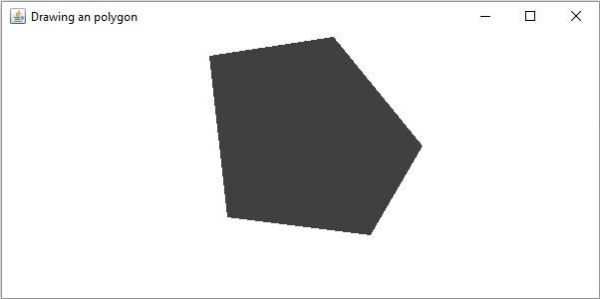# How to draw a polygon in OpenCV using Java?

JavaObject Oriented ProgrammingProgramming

#### Complete Java Programming Fundamentals With Sample Projects

98 Lectures 7.5 hours

#### Get your Java dream job! Beginners interview preparation

85 Lectures 6 hours

#### Core Java bootcamp program with Hands on practice

Featured

99 Lectures 17 hours

A polygon with all the interior angles less than 180 is known as a convex polygon. The org.opencv.imgproc package of Java OpenCV library contains a class named Imgproc. To draw a polygon you need to invoke the fillConvexPoly() method of this class. This method accepts 3 parameters −

• A Mat object representing the image on which the polygon is to be drawn.

• A MatOfPoint object points between which the polygon is to be drawn.

• A Scalar object representing the color of the polygon.

## Example

import org.opencv.core.Core;
import org.opencv.core.Mat;
import org.opencv.core.MatOfPoint;
import org.opencv.core.Point;
import org.opencv.core.Scalar;
import org.opencv.highgui.HighGui;
import org.opencv.imgcodecs.Imgcodecs;
import org.opencv.imgproc.Imgproc;
public class DrawingConvexPolygon {
public static void main(String args[]) {
//Reading the source image in to a Mat object
Mat src = Imgcodecs.imread("D:\images\blank.jpg");
//Drawing an arrowed line
MatOfPoint points = new MatOfPoint (
new Point(108, 71), new Point(232, 52),
new Point(321, 161), new Point(269, 250),
new Point(126, 232), new Point(108, 71)
);
Scalar color = new Scalar(64, 64, 64);
Imgproc.fillConvexPoly (src, points, color);
//Saving and displaying the image
Imgcodecs.imwrite("arrowed_line.jpg", src);
HighGui.imshow("Drawing an polygon", src);
HighGui.waitKey();
}
}

## Output

On executing, the above program generates the following window −